## COMPONENTS FOR LIGHT TRAILERS (Until 3500 kg)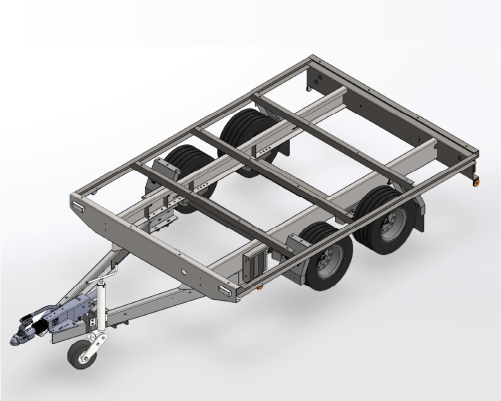WITH FRAME

Approved total weight 2500 kgWe build the frame with the measurements you want

## HOW?

1. See the table below.
2. Take the required measurements (A, B, C, PCD etc…)
3. Send the measurements to us.

### HOW?

1. See the following table.
2. Take the required measures (A, B, C, PCD ecc…)
3. Send us the measures.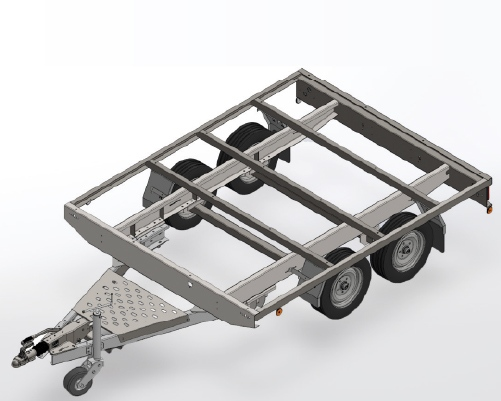WITH FRAME

Approved total weight 2500 kg.We build the frame with the measurements you want

## HOW?

1. See the table below.
2. Take the required measurements (A, B, C, PCD etc…)
3. Send the measurements to us.

### HOW?

1. See the following table.
2. Take the required measures (A, B, C, PCD ecc…)
3. Send us the measures.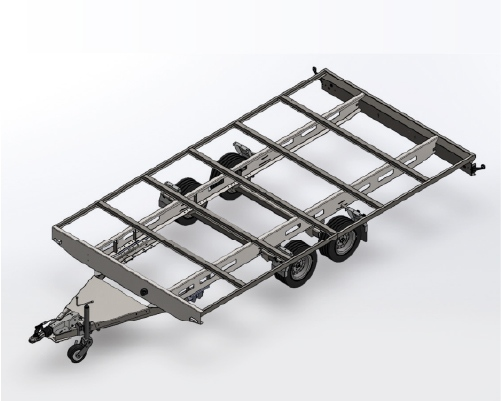WITH FRAME

Approved total weight 3000 kgWe build the frame with the measurements you want

## HOW?

1. See the table below.
2. Take the required measurements (A, B, C, PCD etc…)
3. Send the measurements to us.

### HOW?

1. See the following table.
2. Take the required measures (A, B, C, PCD ecc…)
3. Send us the measures.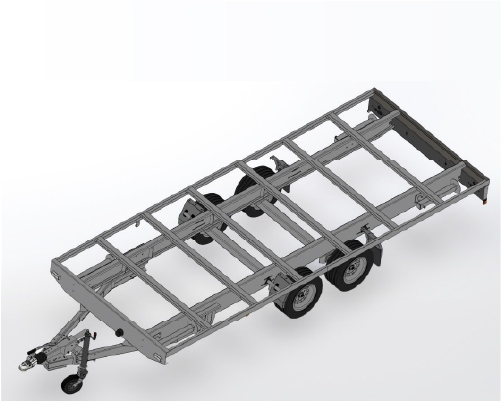WITH FRAME

Approved total weight 3000 kgWe build the frame with the measurements you want

## HOW?

1. See the table below.
2. Take the required measurements (A, B, C, PCD etc…)
3. Send the measurements to us.

### HOW?

1. See the following table.
2. Take the required measures (A, B, C, PCD ecc…)
3. Send us the measures.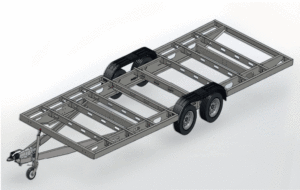WITH FRAME

Approved total weight 3000 kg### HOW?

1. See the following table.
2. Take the required measures (A, B, C, PCD ecc…)
3. Send us the measures.